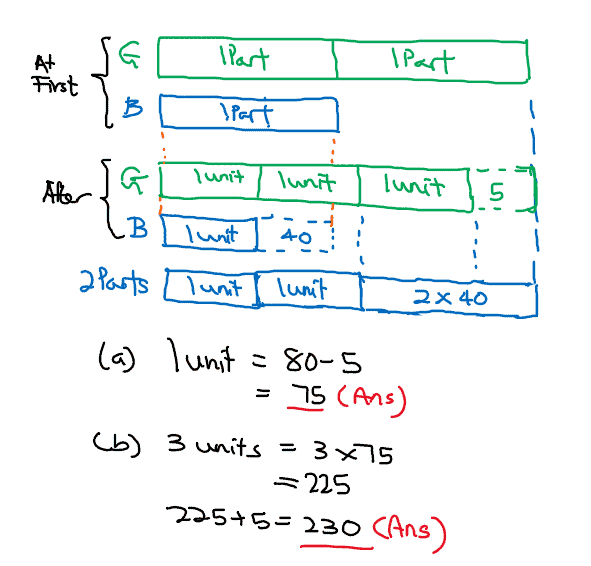# Question

a) How many blue beads did she have left?

b) How many green beads did she have at first?

You do not need to draw a model if you understand the concept.

At first——-G:B=2u:1u

Now———-G:B=3p:1p

2u-5=3p OR 2u-3p=5

1u-40=1p OR 1u-1p=40

1u-1p=40 x2 =2u-2p=80

If 2u-3p=5, and 2u-2p=80, 80-75 is 1p!

So,80-75=5(1p)(a)

75 x 3 =225

225+5=230(b)

Hope you understand!